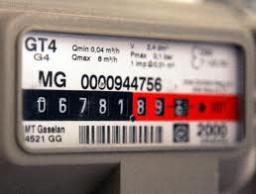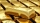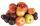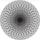# Rates

When gas consumption, the consumer may choose one of two rates:
1. rate A - which pays 0.4 € per 1 m3 of gas a flat monthly fee of 3.9 € (regardless of consumption)
2. rate B - which pays 0.359 € per 1 m3 of gas a flat monthly fee of 12.5 €

From what monthly consumption is more convenient for the customer rate B?

Correct result:

x =  209.8 m3

#### Solution:

$\ \\ 0.4 x + 3.9 > 0.359 x + 12.5 \ \\ \ \\ x > \dfrac{ 12.5-3.9 } { 0.4-0.359 } \ \\ x > 209.8 \ \text{m}^3$Our examples were largely sent or created by pupils and students themselves. Therefore, we would be pleased if you could send us any errors you found, spelling mistakes, or rephasing the example. Thank you!

Leave us a comment of this math problem and its solution (i.e. if it is still somewhat unclear...):ThomasDr Math
we think that maybe "hacking mathematics" or "play with mathematics". All relevant domains is occupied.## Next similar math problems:

• Precious metalsIn 2006-2009, the value of precious metals changed rapidly. The data in the following table represent the total rate of return (in percentage) for platinum, gold, an silver from 2006 through 2009: Year Platinum Gold Silver 2009 62.7 25.0 56.8 2008 -41.3
• Rate or interestAt what rate percent will Rs.2000 amount to Rs.2315.25 in 3 years at compound interest?
• RootThe root of the equation ? is: ?How much and how many times is 72.1 greater than 0.00721?
• TimeWrite time in minutes rounded to one decimal place: 5 h 28 m 26 s.
• 1 page1 page is torn from the book. The sum of the page numbers of all the remaining pages is 15,000. What numbers did the pages have on the page that was torn from the book?
• Evaluate - order of opsEvaluate the expression: 32+2[5×(24-6)]-48÷24 Pay attention to the order of operation including integers
• The ticketsThe tickets to the show cost some integer number greater than 1. Also, the sum of the price of the children's and adult tickets, as well as their product, was the power of the prime number. Find all possible ticket prices.
• Three members GPThe sum of three numbers in GP (geometric progression) is 21 and the sum of their squares is 189. Find the numbers.
• Expression 8Evaluate this expressions: a) 5[3 + 4(2.8 - 3)] b) 5×(8-4)÷4-2Add marks (+, -, *, /, brackets) to fullfill equations 1 3 6 5 = 10 This is for the 4th grade of the primary school - with no negative numbers yetVolume of the cube is 216 cm3, calculate its surface area.Grower harvested 190 kg of apples. Pears harvested 10 times less. a) How many kg pears harvested? b) How many apples and pears harvested? c) How many kg harvested pears less than apples?The division of numbers 18 and 6 increase by product of the numbers 156 and 0.001How many times is number 56 larger than number 46?The prism with the edges of the lengths x cm, 2x cm and 3x cm has volume 20250 cm3. What is the area of surface of the prism?Three metal balls with volumes V1=71 cm3 V2=78 cm3 and V3=64 cm3 melted into one ball. Determine it's surface area.